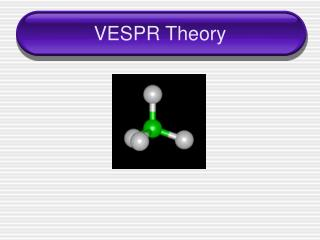Download PresentationVESPR Theory

# VESPR Theory - PowerPoint PPT PresentationDownload Presentation## VESPR Theory

- - - - - - - - - - - - - - - - - - - - - - - - - - - E N D - - - - - - - - - - - - - - - - - - - - - - - - - - -
##### Presentation Transcript

1. VESPR Theory

2. Molecular Structure • Molecular structure – _______________ arrangement of atoms in a molecule

3. VSEPR Theory • VSEPR Theory ________________________ • The structure around a given atom is determined by minimizing the _______________ • The electrons and elements bonded to the central atom want to be _______________ as possible

4. VSEPR Steps • Draw the Lewis structure for the molecule • Count the total number of things that are around the central atom to determine the electron pair geometry • Imagine that the lone pairs of electrons are invisible and describe the molecular shape

5. SORRY… • Yes…you must memorize the main shapes and bond angles

6. 2 Electron Pairs • If there are 2 things attached to the central atom, the shape is linear • Bond angle = 180°

7. 3 Electron Pairs • If there are 3 electron pairs the shape will be trigonal planar • Bond angle = 120°

8. 3 electron pairs • Now imagine that you have 3 electron pairs, but one is just a lone pair (invisible) what would it look like then?

9. 4 electron pairs • If there are 4 electron pairs, the shape will be tetrahedral • Bond angle = 109.5°

10. 4 electron pairs • What if 1 of the electron pairs is a lone pair (invisible)? What would it look like then? • Trigonal Pyramidal

11. 4 electron pairs • What if there are 2 lone pairs (invisible)? What would it look like then? • bent

12. 5 electron pairs • If there are 5 electron pairs the shape will be Trigonal Bipyramidal • Bond angles = 90º & 120º

13. 5 electron pairs • What is there is 1 lone pair (invisible) • Seesaw

14. 5 electron pairs • What is there are 2 lone pairs (invisible) • T-shaped

15. 6 electron pairs • If there are 6 electron pairs the shape will be octahedral • Bond angle = 90°

16. 6 electron pairs • What if there is 1 lone pair (invisible)? • Square pyramidal

17. 6 electron pairs • What if there are 2 lone pairs (invisible) • Square planar